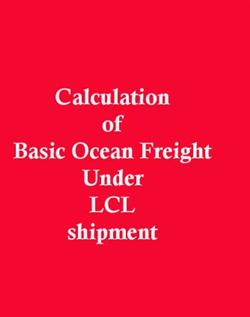## How to Compute Basic Ocean freight under LCL shipment?How to calculate Chargeable Volume?

How to find chargeable volume under LCL export with examples?

Calculation of basic Ocean Freight under LCL cargo is on the basis of per CBM (Cubic Meter) rate or per Ton (per 1000 kg’s gross weight) whichever is higher.

Or it can be also stated as, basic ocean freight under LCL shipment is calculated on the basis of chargeable volume of cargo.

How to calculate chargeable volume of cargo?  Chargeable volume means total cubic meter volume of cargo or total gross weight in Tons whichever is higher.

More examples based on ‘Gross weight’ are accounted in for finding chargeable volume to calculate total ocean freight.

EXAMPLES 1

Find the ocean freight for the following LCL shipment. Freight charge is \$ 40 per CBM. Measurement of cargo is given as,

Length - 60cm

Width - 1.5m

Height - 2m

Weight - 3000 kg

Solution

Volume  =length *weight*height

=0.6*1.5*2

=1.8 CBM

Since 3000 kg is equal to 3 CBM

Calculation of basic Ocean Freight under LCL cargo is on the basis of per CBM (Cubic Meter) rate or per Ton (per 1000 kg’s gross weight) whichever is higher.

1.8< 3

i.e, 3*40= \$ 120

Total ocean freight for the LCL shipment =\$ 120

Gross weight is accounted

Example 2:

Find ocean freight for the following LCL shipment .Ocean freight is 55\$ per cbm. There are 4 parcels and each parcel is measuring 2 m length, 75 cm width, and 70cm height. Gross weight is mentioned as 10 tons.

Solution:

Length           –  2m

Width            –   75cm (0.75m)

Height           – 70cm (0.7m)

No. of Parcel  – 4

Rate             -   \$ 55 per cbm

Weight          - 10 tons

V            =   L* W* H

= 2*0.75*0.7

=1.05 CBM

i.e,     1.05*4

=4.2 CBM

Weight is 10 tons, so it considered as 10 CBM

Basic Ocean Freight under LCL cargo is calculated on the basis of per CBM (Cubic Meter) rate or per Ton (per 1000 kgs gross weight) whichever is higher.

So,      10 > 4.2

à      10*55 = 550

Total ocean freight for the LCL shipment = \$ 550

Gross weight is accounted

EXAMPLES 3

Find the total ocean freight for the following LCL shipment. Freight charges are quoted as \$40 per cbm. Gross weight is 1500 kg. Measurement of cargo is given as,

Length    =  40 cm (0.4 m)

Width     =  1.3 m

Height    = 1.8m

Weight    = 1500 kg (equals to 1.5 cbm)

Solution

V    = L* W* H

= 0.4* 1.3*1.8

= 0.936 cbm

Basic Ocean Freight under LCL cargo is calculated on the basis of per CBM (Cubic Meter) rate or per Ton (per 1000 kgs gross weight) whichever is higher. Here,

0.936 < 1.5

So, 1.5*40 = \$60

Gross weight is accounted

How to Choose a product for export business

How to send export samples to foreign buyer? Tips to send samples to foreign buyer

Export marketing : How to get order

Disadvantages of Letter of credit (LC) for Exporter

How to import to India from Guinea?

How to export from India to Guinea?

Documents required for Export from Guinea

Customs procedures for Guinea Export

Registration required to export from Guinea

Documents needed for Guinea import

How to claim IGST refund on exports?

Export procedure changes after GST implementation

Factory stuffing procedures after GST implementation

Import of goods attracts IGST but not CVD under GST regime

Import goods attracts IGST and CVD under GST regime

Import Goods attract IGST, CVD and Compensation Cess under GST regime

Safeguard duty and Anti-dumping duty after GST implementation

What is Sin or De-Merit Goods under GST

What is Show cause notice under GST

What is SGST Law under GST

Term SGST under GST

What is apportionment of tax and settlement of funds under GST